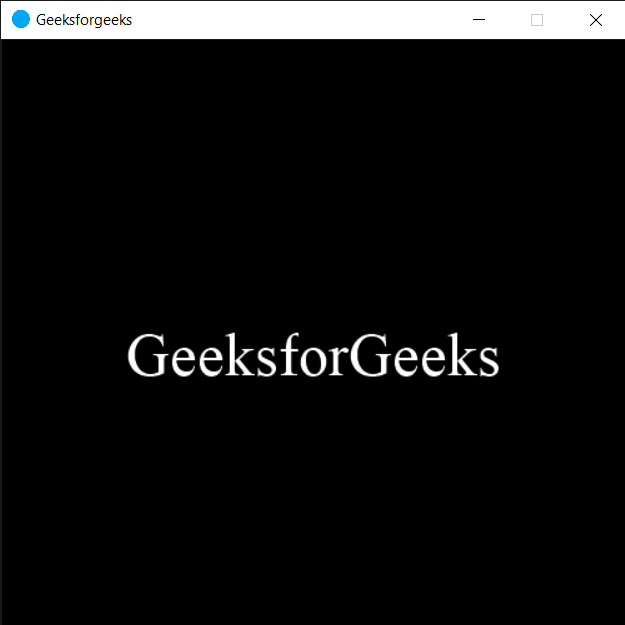Open in App
Not now

# PYGLET – On Key Press Event

• Last Updated : 30 Jul, 2021

In this article we will see how we can trigger on key press event in PYGLET module in python. Pyglet is easy to use but powerful library for developing visually rich GUI applications like games, multimedia etc. A window is a “heavyweight” object occupying operating system resources. Windows may appear as floating regions or can be set to fill an entire screen (fullscreen). A key on the keyboard was pressed (and held down). In pyglet 1.0 the default handler sets has_exit to True if the ESC key is pressed. Key press event is the major event to handle keyboard inputs.
We can create a window with the help of command given below

`pyglet.window.Window(width, height, title)`

Below is the syntax of the on key press event, this method get called when this event is triggered  :

```@window.event
def on_key_press(symbol, modifiers):
print("Key is pressed")```

Example : Below is the implementation

## Python3

 `# importing pyglet module``import` `pyglet``import` `pyglet.window.key`` ` `# width of window``width ``=` `500`` ` `# height of window``height ``=` `500`` ` `# caption i.e title of the window``title ``=` `"Geeksforgeeks"`` ` `# creating a window``window ``=` `pyglet.window.Window(width, height, title)`` ` `# text ``text ``=` `"GeeksforGeeks"`` ` `# creating a label with font = times roman``# font size = 36``# aligning it to the centre``label ``=` `pyglet.text.Label(text,``                          ``font_name ``=``'Times New Roman'``,``                          ``font_size ``=` `36``,``                          ``x ``=` `window.width``/``/``2``, y ``=` `window.height``/``/``2``,``                          ``anchor_x ``=``'center'``, anchor_y ``=``'center'``)`` ` `new_label ``=` `pyglet.text.Label(text,``                          ``font_name ``=``'Times New Roman'``,``                          ``font_size ``=` `10``,``                          ``x ``=` `25``, y ``=` `25``)`` ` `# on draw event``@window``.event``def` `on_draw():    `` ` `     ` `    ``# clearing the window``    ``window.clear()``     ` `    ``# drawing the label on the window``    ``label.draw()`` ` `     ` `# key press event    ``@window``.event``def` `on_key_press(symbol, modifier):``     ` `    ``print``(``"Some key is pressed"``)``     ` `    ``# key "C" get press``    ``if` `symbol ``=``=` `pyglet.window.key.C:``         ` `        ``print``(``"Key C is pressed"``)`` ` ` ` `# on hide event``@window``.event``def` `on_hide():``     ` `    ``# printing some message``    ``print``(``"Window is minimized"``)``     ` ` ` `  ` ` ` `# image for icon``img ``=` `image ``=` `pyglet.resource.image(``"logo.png"``)`` ` `# setting image as icon``window.set_icon(img)`` ` `                ` `# start running the application``pyglet.app.run()`

Output :```Some key is pressed
Key C is pressed```

My Personal Notes arrow_drop_up